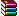# 【Access示例】判断是否为小数

'判断一个数字是否为小数
'IsDecimal(需要进行判断的数字)
'返回值为True 代表是小数 反之为整数

Public Function IsDecimal(num As Double)
If num = Round(num) Then
IsDecimal = False
Else
IsDecimal = True
End If
End Function

'具体使用示例

Function test()
Dim num As Double
num = 1.7
If
IsDecimal(num) Then
MsgBox num & " 是小数"
End If

End Function点击下载此附件

Access软件网QQ交流群 (群号:198347485) 　　　　　　access源码网店

## 发表评论您的评论将提升作者分享的动力！快来评论一下吧！

 用户名： 密　码： 内　容：

## 最新帖子